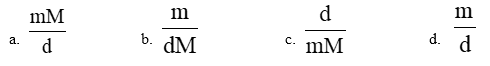# Problem: If m represents the number of moles of a substance, M represents the molar mass of the substance, and d represents the density of the substance, which of the following expressions equals the volume of the sample substance?

###### Problem Details

If m represents the number of moles of a substance, M represents the molar mass of the substance, and d represents the density of the substance, which of the following expressions equals the volume of the sample substance?Frequently Asked Questions

What scientific concept do you need to know in order to solve this problem?

Our tutors have indicated that to solve this problem you will need to apply the SI Units concept. You can view video lessons to learn SI Units. Or if you need more SI Units practice, you can also practice SI Units practice problems.

What is the difficulty of this problem?

Our tutors rated the difficulty ofIf m represents the number of moles of a substance, M repres...as medium difficulty.

How long does this problem take to solve?

Our expert Analytical Chemistry tutor, Jules took 2 minutes and 23 seconds to solve this problem. You can follow their steps in the video explanation above.